# Electronics and Communication Engineering - Exam Questions Papers

1.
Determine the potential difference between the points A and B in the steady state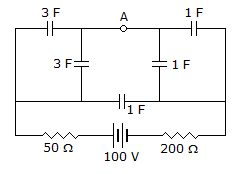40 V
60 V
25 V
75 V
Explanation: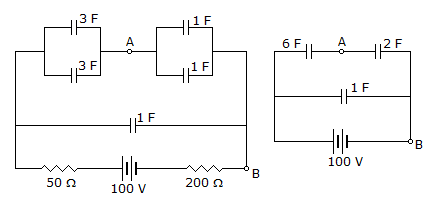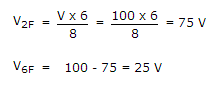2.
Find the value of K and velocity constant Kv so that the maximum overshoot in the unit step response is 0.2 and the peak time is 1 sec.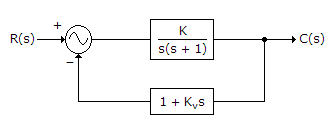12.37, 0.175
12.86, 2.175
11.36, 1.175
1.86, 0.175
Explanation: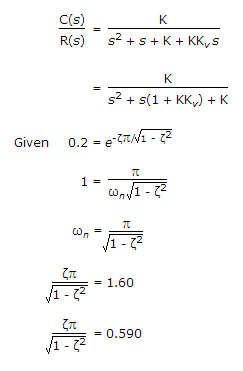ζ2 = 0.259(1 - ζ2) = 0.259 - 0.259ζ2

1.259ζ2 = 0.259

ζ2 = 0.206

ζ = 0.45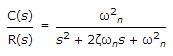ω2n = K

ωn = K and 2ζωn = 1 + KKv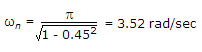ωn = K

K = ω2n

K = 12.37

1 + 12.37Kv = 2 x 0.45 x 3.52

Kv = 0.175 .

3.
The transfer function of a discrete time LTI system is given by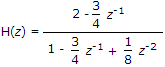Consider the following statements:
S1: The system is stable and causal for ROC: |z| > 1/2
S2: The system is stable but not causal for ROC: |z| < 1/4
S3: The system is neither stable nor causal for ROC: 1/4 < |z| < 1/2
Which one of the following statements is valid?
Both S1 and S2 are true
Both S2 and S3 are true
Both S1 and S3 are true
S1, S2 and S3 are all true
Explanation:

S1 true, S2 False (meter stable nor causal) S3 True.

4.
In the following network, the switch is closed at t = 0- and the sampling starts from t = 0. The sampling frequency is 10Hz.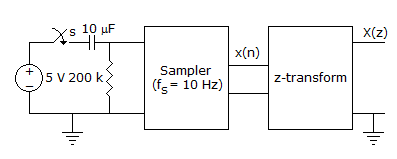The expression and the region of convergence of the z-transform of the sampled signal are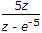|z| < e-5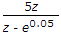|z| < e-0.05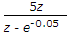|z| < e-0.05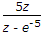|z| > e-5
Explanation:

X(Z) =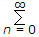5.e-0.05n.Z-n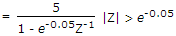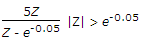.

5.
The logic function implemented by the following circuit at the terminal OUT is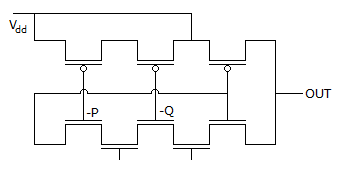P NOR Q
P NAND Q
P OR Q
P AND Q